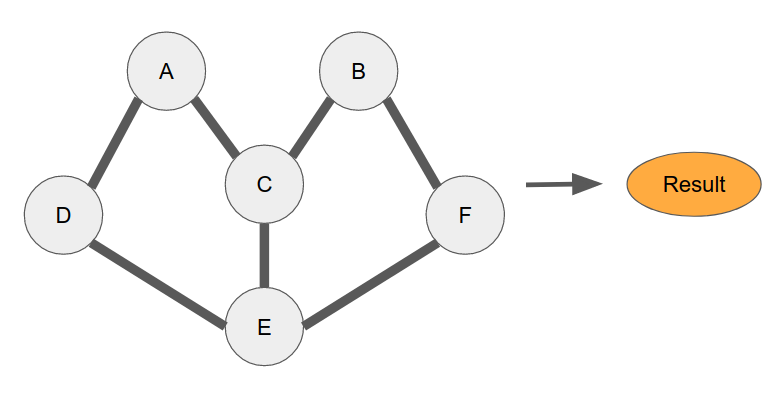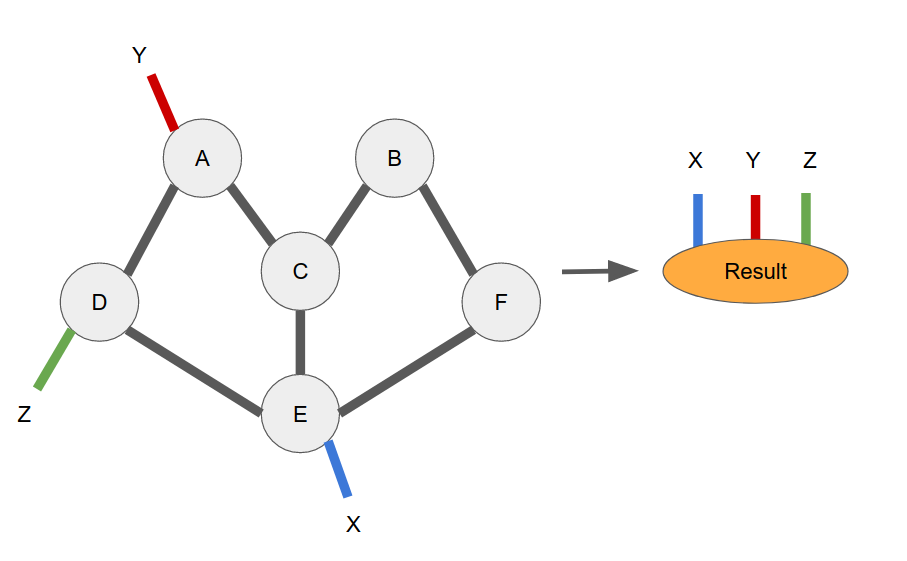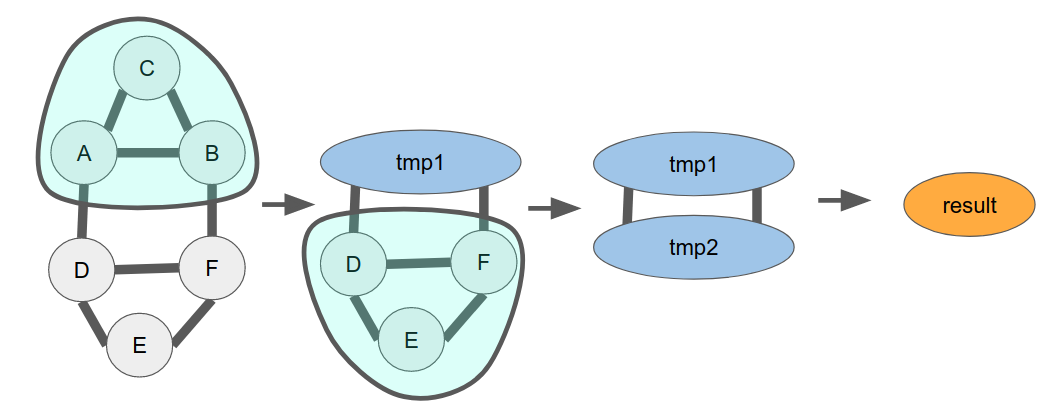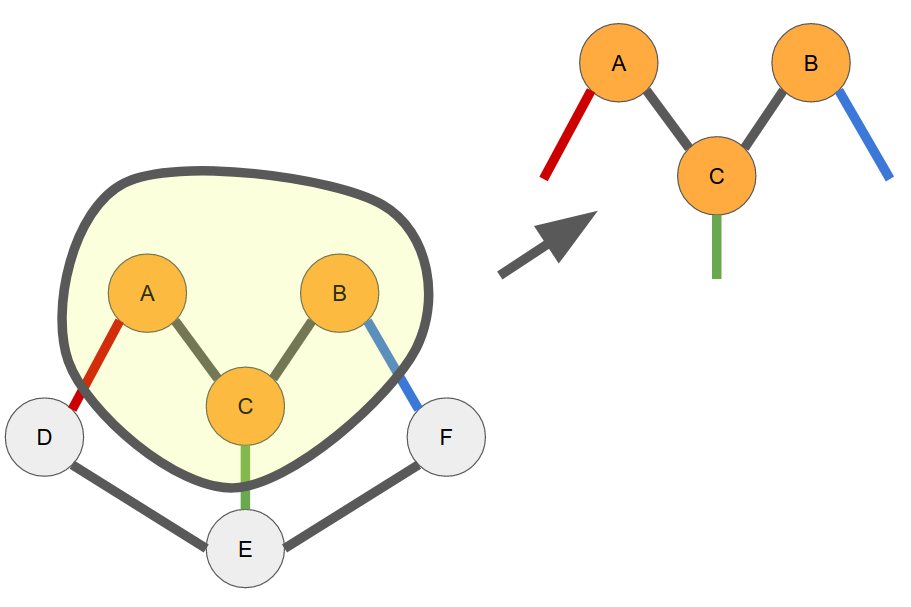# Copying and Contracting¶

## Automated contraction¶

Finding good contracting paths for arbitrary tensor networks can be challenging. In fact, finding the optimal contration path is proven to be NP-Hard. While we can’t crack NP hard problems, we can get decent results for small sized systems.

Using a contraction algorithm is very easy.

result = tn.contractors.auto([a, b, c, d, e, f])We have several contraction algorithms avaliable as of April 2020.

• optimal: Find the true optimal path via brute force. It can be extremly slow for more than ~10 nodes.
• greedy: Continuously do the cheapest contraction possible. Works well as a default for networks with many nodes.
• branch: Brute search, but only check the top n possiblities per step.
• auto: Automatically decide which of the above 3 algorithms to use.

When contracting a network with more than one dangling leg, you must specify the output order of the dangling legs.

result = tn.contractors.auto(
[a, b, c, d, e, f]
output_edge_order=[x, y, z]
)If you do not care about the final output order (for instance, if you are only doing a partial network contraction and the intermidiate order doesn’t matter), then you can set ignore_edge_order=True and you won’t need to supply an output_edge_order.

## Contracting subgraph¶

There are many instances when you want to contract only a subset of your network. Perhaps you know good intermidiate states, but not how to get there efficiently. You can still very easily get a good contraction order by using the subnetwork contraction feature of the contractors.

# Say all of these nodes are connected somehow.
a, b, c, d, e, f = build_your_network(...)
tmp1  = tn.contractors.optimal([a, b, c], ignore_edge_order=True)
tmp2 = tn.contractors.optimal([d, e, f], ignore_edge_order=True)
result = tmp1 @ tmp2## Reusing networks¶

When building tensor networks, it’s very common to want to use a single tensornetwork for many purposes. For example, a user may want to use an MPS to calculate an inner product with some product state as well as its partition function. Nodes within tensornetwork are mutable, so to presever the original MPS connectivity, you’d need to copy the nodes before contraction.

# Calcualte the inner product of two MPS/Product state networks.
def inner_product(x: List[tn.Node], y: List[tn.Node]) -> tn.Node:
for a, b in zip(x, y)
# Assume all of the dangling edges are mapped to the name "dangling"
tn.connect(a["dangling"], b["dangling"])
return tn.contractors.greedy(x + y)

mps_nodes = build_your_mps(...)

# Calculate the inner product with a product_state
product_state_nodes = build_your_product_state(...)
mps_copy = tn.replicate_nodes(mps_nodes)
result1 = inner_product(mps_copy, product_state_nodes)

# Calculate the partition function.
mps_copy = tn.replicate_nodes(mps_nodes)
mps_conj_copy = tn.replicate_nodes(mps_nodes, conjugate=True)
result2 = inner_product(mps_copy, mps_conj_copy)


If you need more advance access to your copied network, tn.copy will return two dictionaries mapping your original nodes and edges to the newly copied versions.

## Copying subnetwork¶

When copying a subgraph of a network, all of the connections between nodes are preserved in the copied network. Any standarded edges between two nodes where one is copied and isn’t, that edge becomes a dangling edge on the same axis of the copied node.

# Say all of these nodes are connected somehow.
a, b, c, d, e, f = build_your_network(...)
new_a, new_b, new_c = tn.replicate_nodes([a, b, c])